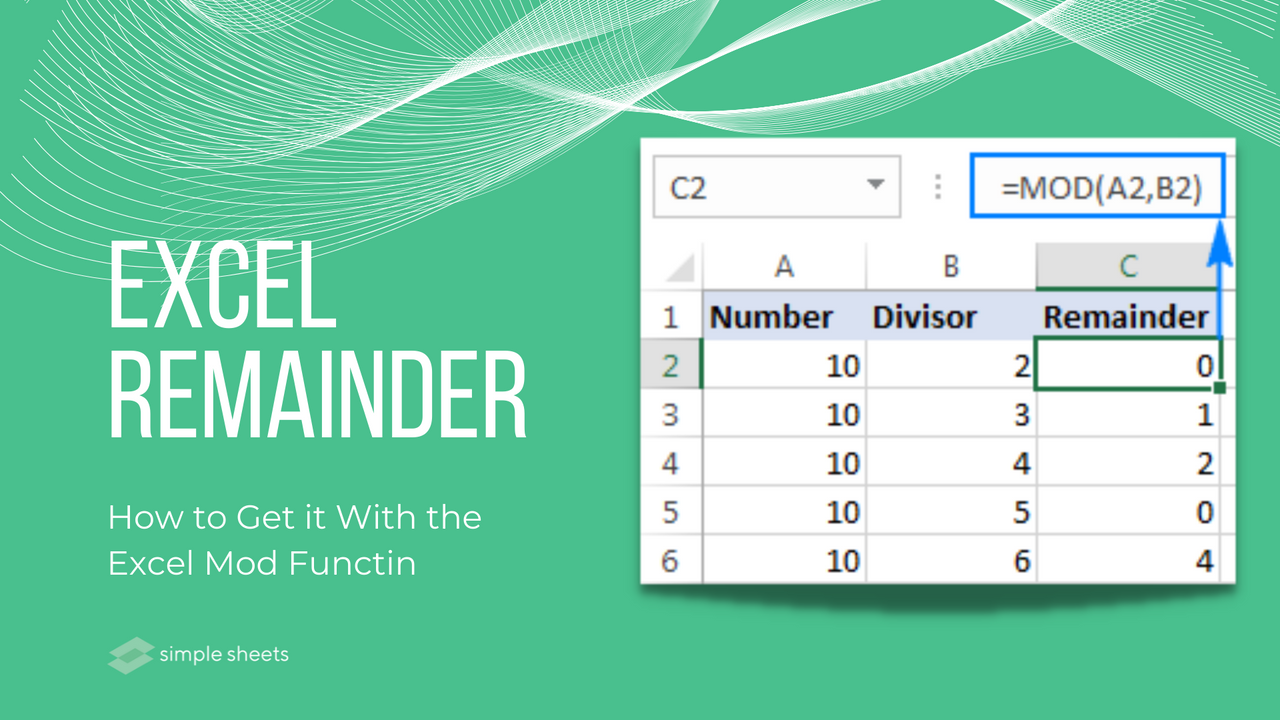# Excel Remainder: How to Get it With the Excel Mod Function

Jul 26, 2022

If you use Microsoft Excel, often you will likely know how easy it is to divide two numbers.

However, what do you need to do to get the remainder from a division within Excel?

Fortunately, the MOD function in Excel can achieve this calculation with ease.

This article will explain the MOD function in more detail and why it is one of the most important excel functions you have at your disposal.## How Does the MOD Function Work?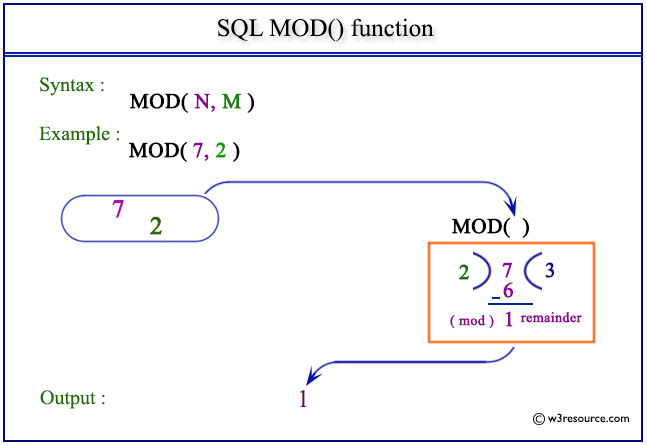The role of the MOD function is to return the remainder after division. The MOD function syntax takes two separate arguments. First, the Number is the number divided, and the divisor is the number that gets used to divide.

You need both function arguments to use the MOD function correctly. Otherwise, the MOD formula will not work and will return #VALUE!.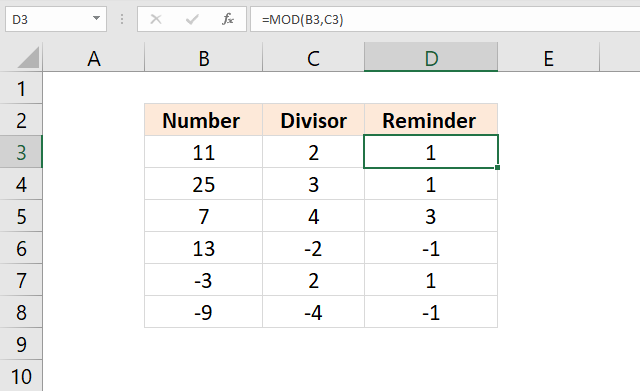### Equation

The result offered by the MOD function is calculated in the below equation:

=n-d*INT(n/d)

In this equation, the n is the number, and the d is the divisor. INT represents the INT function. This MOD formula can lead to unexpected results sometimes due to how the INT function rounds any negative numbers down.### Negative numbers

The numeric value from MOD offers the same sign as the divisor.

If your divisor is positive, the MOD result will be positive. If the divisor is negative, the MOD result will be negative.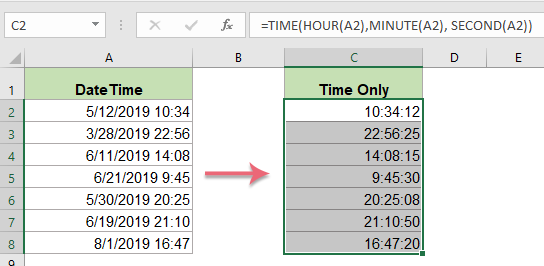### Time from DateTime

Another way to use the mod function other than for conditional formatting, to calculate cells, and highlight cells, to name a few examples, is to extract the time value of an Excel date, so long as it includes DateTime.

### Large numbers

With large numbers, you might notice the MOD function returns a #NUM error. If this is the case, and you still want to execute a formula that returns the remainder, you should use the following formula.

=number-(INT(number/divisor)*divisor)

## Excel Remainder: Summary and Key Takeaways

Now you know how to get the remainder in Excel using the MOD function. Now you can add numbers divided into any worksheet or presentation as you please. MOD and Column functions are some of the most important Excel functions.

Remember to keep practising using this built-in function in an example workbook. The more MOD examples you can create following the correct formula, the more confident a MOD number divisor you will become!## Frequently Asked Questions About Mod Function Returns

### Is there a remainder function in Excel?

The MOD function within Excel is the tool you need to use to find the remainder after the division of one number by another, as highlighted in this Microsoft Excel tutorial.

### How do you separate QUOTIENT and remainder in Excel?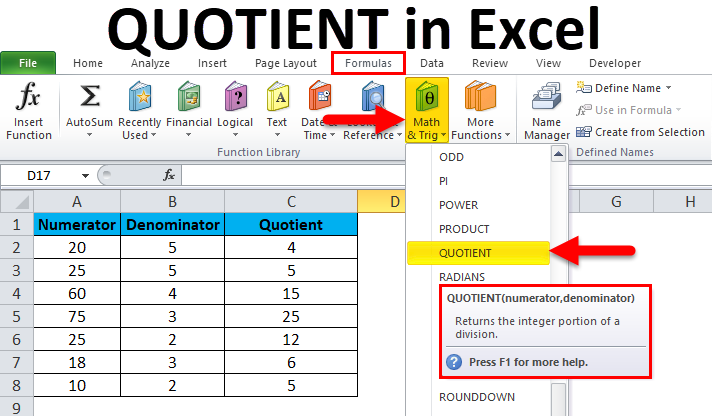In Excel, it is common practice to use the division operator forward slash to return a decimal number.

The QUOTIENT formula only returns the integer portion and not the decimal number.

### What does MOD mean in Excel formula?

The Excel MOD function is used to return the remainder of a division between two numbers.

## Related Articles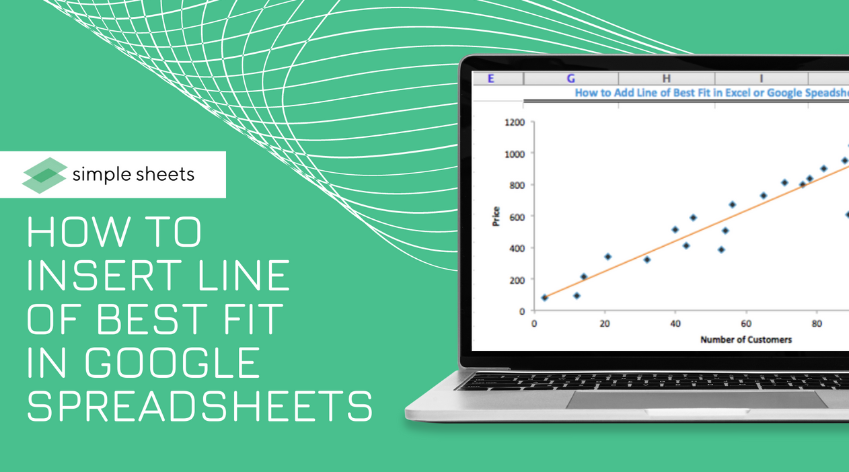How to Insert Line of Best Fit in Google Spreadsheets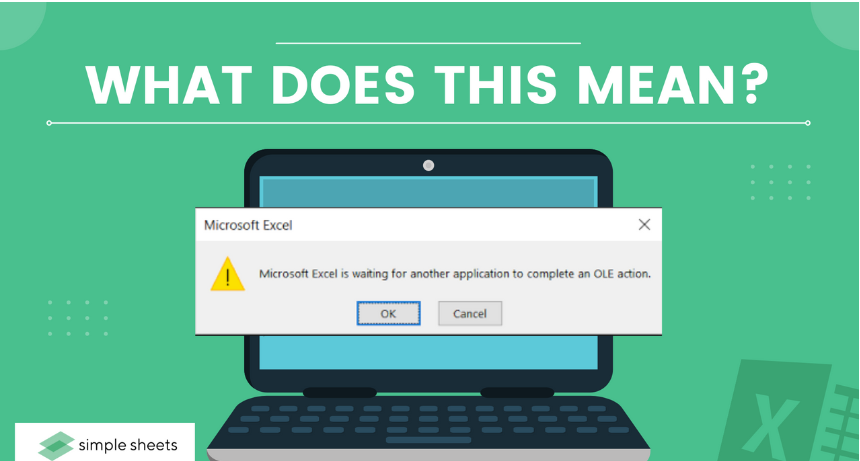Microsoft Excel is Waiting for Another Application to complete an OLE Action - What Does This Mean?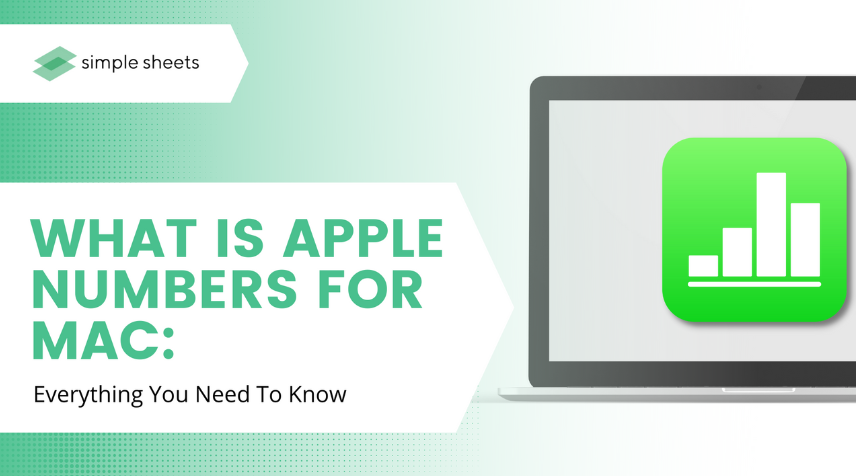What is Apple Numbers For Mac: Everything You Need to Know

Lorem ipsum dolor sit amet, consectetur adipiscing elit. Cras sed sapien quam. Sed dapibus est id enim facilisis, at posuere turpis adipiscing. Quisque sit amet dui dui.

#### Stay connected with news and updates!

Join our mailing list to receive the latest news and updates from our team.
Don't worry, your information will not be shared.

We hate SPAM. We will never sell your information, for any reason.